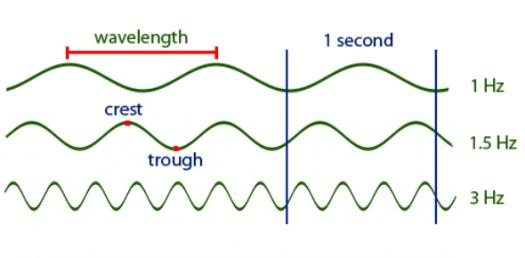# Trivia Quiz: What Do Know About Frequencies And Wavelengths?

15 Questions | Total Attempts: 208SettingsWhat Do Know About Frequencies And Wavelengths? A wavelength is the distance between two waves whereas the frequency is the number of waves that pass a given point at a time. In the quiz below, we shall dig deeper into the relationship between the two and how they are measured. How about you give it a shot and refresh your memory?

• 1.
A long wavelength means:
• A.

Low frequency

• B.

High frequency

• 2.
If the amplitude is high, the sound is:
• A.

Louder

• B.

Quieter

• 3.
This wave represents a sound that is:
• A.

Low-pitched

• B.

High-pitched

• C.

There is no way to tell.

• 4.
Which of these waves has the lowest frequency?
• A.

Red

• B.

Orange

• C.

Green

• D.

Blue

• E.

Purple

• 5.
The symbol λ stands for:
• A.

Frequency

• B.

Wavelength

• C.

Amplitude

• 6.
What is a unit used to measure frequency?
• A.

Meters

• B.

Cycles/second

• C.

Meters/second

• D.

Waves/second

• 7.
If the amplitude is low, the light is:
• A.

Brighter

• B.

Dimmer

• 8.
Microwaves have a higher frequency than X-Rays. True or False?
• A.

True

• B.

False

• 9.
In which situation does sound travel fastest?
• A.

High density medium (like solids)

• B.

Medium density medium (like liquids)

• C.

Low density medium (like gases)

• D.

No medium

• 10.
In which situation does light travel fastest?
• A.

Low density medium (like gases)

• B.

Medium density medium (like liquids)

• C.

High density medium (like solids)

• D.

No medium

• 11.
Sound travels faster than light. True or False?
• A.

True

• B.

False

• 12.
Which is a CORRECT formula relating to wavelength, frequency, and speed of waves?
• A.

V = λ➗⨏

• B.

λ = v●⨏

• C.

λ = v➗⨏

• D.

⨏ = λ●v

• 13.
Which electromagnetic energy is most dangerous and why?
• A.

Gamma Waves -> Lowest frequency

• B.

Infrared -> Highest frequency

• C.

Gamma waves -> Highest frequency

• D.

X-rays -> Highest energy

• 14.
Blue light has more energy than orange light. True or False?
• A.

True

• B.

False

Related TopicsBack to top